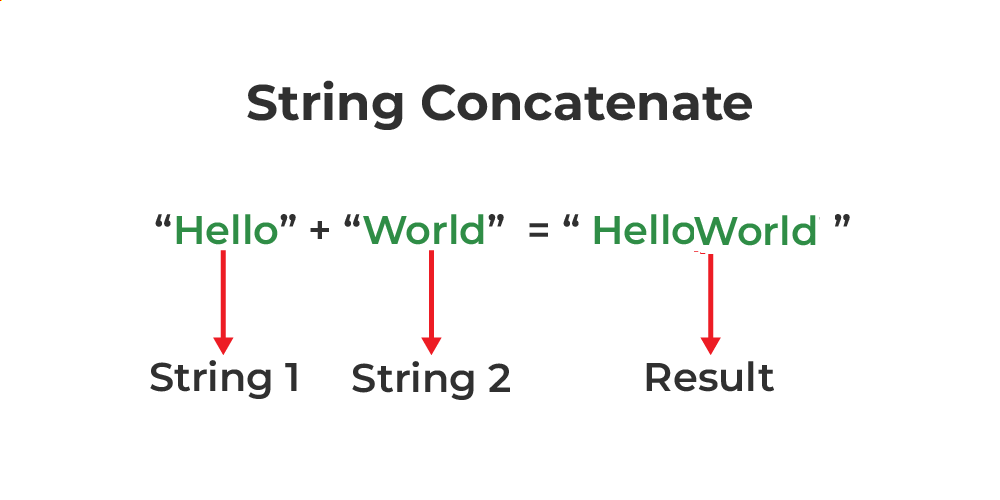# String Concatenation in C++

The string is a type of data structure used for storing characters. Concatenating strings in C++ is one of the most discussed topics related to strings. There are multiple methods to concat strings using user-defined methods, and a couple of methods for the concatenation of strings using pre-defined methods. Let’s check on all of these methods.## Methods of Concatenate String

There are 6 methods to Concatenate String  as mentioned below:

1. Using append( ) Function.
2. Using ‘+’ Operator.
3. Using strcat( ) Function.
4. Using C++ for Loop.
5. Using Inheritance.
6. Using the Friend Function and strcat() Function.

### 1. Using append() Function

The append() function is a member function of the std::string class. Using this function, we can concatenate two std::string objects (C++ style strings) as shown in the below example.

Syntax:

`string& string::append (const string& str);Here,str: String to be appended.`

Below is the C++ program for string concatenation using the append() function:

## C++

 `// C++ Program for string` `// concatenation using append` `#include ` `using` `namespace` `std;`   `// Driver code` `int` `main()` `{` `    ``string init(``"this is init"``);` `    ``string add(``" added now"``);`   `    ``// Appending the string.` `    ``init.append(add);`   `    ``cout << init << endl;` `    ``return` `0;` `}`

Output

```this is init added now
```

### 2. Using ‘+’ Operator

This is the easiest method for the concatenation of two strings. The + operator adds strings and returns a concatenated string. This method only works for C++ style strings (std::string objects) and doesn’t work on C style strings (character array).

Syntax:

`string new_string = init + add;`

Below is the C++ program for string concatenation using ‘+’ operator:

## C++

 `// C++ Program for string` `// concatenation using '+' operator` `#include ` `using` `namespace` `std;`   `// Driver code` `int` `main()` `{` `    ``string init(``"this is init"``);` `    ``string add(``" added now"``);`   `    ``// Appending the string.` `    ``init = init + add;`   `    ``cout << init << endl;` `    ``return` `0;` `}`

Output

```this is init added now
```

### 3. Using strcat( ) Function

The C++ strcat( ) function is a built-in function defined in <string.h> header file. This function concatenates the two strings init and add and the result is stored in the init string. This function only works for C-style strings (character arrays) and doesn’t work for C++-style strings (std::string objects).

Syntax:

`char * strcat(char * init, const char * add);`

Below is the C++ program for string concatenation using strcat() function:

## C++

 `// C++ Program for string` `// concatenation using strcat` `#include ` `#include ` `using` `namespace` `std;`   `// Driver code` `int` `main()` `{` `    ``char` `init[] = ``"this is init"``;` `    ``char` `add[] = ``" added now"``;`   `    ``// Concatenating the string.` `    ``strcat``(init, add);`   `    ``cout << init << endl;`   `    ``return` `0;` `}`

Output

```this is init added now
```

### 4. Using  for Loop

Using a loop is one of the most basic methods of string concatenation. Here, we are adding elements one by one while traversing the whole string and then another string.  The final result will be the concatenated string formed from both strings.

Below is the C++ program for string concatenation using for loop:

## C++

 `// C++ Program for string` `// concatenation using for loop` `#include ` `using` `namespace` `std;`   `// Driver code` `int` `main()` `{` `    ``string init(``"this is init"``);` `    ``string add(``" added now"``);`   `    ``string output;`   `    ``// Adding element inside output` `    ``// from  init` `    ``for` `(``int` `i = 0; init[i] != ``'\0'``; i++) ` `    ``{` `        ``output += init[i];` `    ``}`   `    ``// Adding element inside output` `    ``// fromt add` `    ``for` `(``int` `i = 0; add[i] != ``'\0'``; i++) ` `    ``{` `        ``output += add[i];` `    ``}`   `    ``cout << output << endl;` `    ``return` `0;` `}`

Output

```this is init added now
```

### 5. Using Inheritance

Below is the C++ program for string concatenation using inheritance:

## C++

 `// C++ program for string concatenation ` `// using inheritance` `#include   ` `#include   ` `using` `namespace` `std;  ` `  `  `// Base class  ` `class` `base  ` `{  ` `    ``protected``:  ` `        ``virtual` `string concatenate(string &str1, ` `                                   ``string &str2) = 0;  ` `};    ` `  `  `// Derive class` `class` `derive: ``protected` `base {  ` `    ``public``:  ` `        ``string concatenate (string &str1, ` `                            ``string &str2) ` `        ``{  ` `            ``string temp;  ` `            ``temp = str1 + str2;  ` `            ``return` `temp;  ` `        ``}  ` `};  ` `  `  `// Driver code` `int` `main()  ` `{  ` `    ``string init(``"this is init"``);` `    ``string add(``" added now"``);   ` `      `  `    ``// Create string object  ` `    ``derive obj;  ` `      `  `    ``// Print string  ` `    ``cout << obj.concatenate (init, add);  ` `      `  `    ``return` `0;  ` `}`

Output

```this is init added now
```

### 6. Using the Friend Function and strcat() function

Below is the C++ program for string concatenation using the friend function and strcat() function:

## C++

 `// C++ program for string concatenation` `// using friend function and strcat() ` `#include   ` `#include   ` `using` `namespace` `std;  `   `// Base class   ` `class` `Base {  ` `    ``public``:  ` `      ``char` `init = ``"this is init"``;` `      ``char` `add = ``" added now"``; ` `              `  `      ``friend` `void` `myfun(Base b);        ` `};  ` `  `  `void` `myfun (Base b)  ` `{  ` `    ``// Pass parameter to concatenate ` `    ``strcat` `(b.init, b.add);  ` `      `  `    ``cout << b.init;  ` `}  `   `// Driver code` `int` `main()  ` `{  ` `  `  `    ``// Create object of base class    ` `    ``Base b;  ` `        `  `    ``// pass b object to myfun() to print ` `    ``// the concatenated string  ` `    ``myfun(b); ` `      `  `    ``return` `0;  ` `}`

Output

```this is init added now
```

Whether you're preparing for your first job interview or aiming to upskill in this ever-evolving tech landscape, GeeksforGeeks Courses are your key to success. We provide top-quality content at affordable prices, all geared towards accelerating your growth in a time-bound manner. Join the millions we've already empowered, and we're here to do the same for you. Don't miss out - check it out now!

Previous
Next